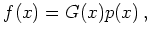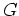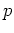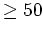Previous: 3.2.3 Diffusion Closure Up: 3. Highest Order Moment Next: 3.4 Gaussian Invariant Closure

# 3.3 Higher Order Statistics

There are no generally applicable methods for estimating a distribution from moments without additional assumptions. For weakly non-normal distributions two formalisms are in widespread use: the cumulant or Edgeworth expansion [Hal92] and the quasi-moment or Gram-Charlier expansion [Hal98]. Both expansions are of the form(3.17)

wheredenotes a Gaussian-like distribution andis a polynomial. To achieve a reasonably good approximation a large number of moments has to be included in the expansion due to the polynomial nature of.

The Gram-Charlier series is merely a kind of Fourier expansion in the set of Hermite polynomials which diverges in many situations of practical interest [BM98]. The Edgeworth expansion gives an asymptotic series.

Both Gram-Charlier and Edgeworth expansion can result in distribution functions which take on negative values. With positivity constraints the domain of skewness and kurtosis which can be represented is smaller than for the full maximum entropy approximation [RJ02].

Expansions which use similar classes of ansatz functions are used in the spherical harmonic expansion [Str00], [BAD96], in Grad's expansion and in various Galerkin approaches [RSZ01], [SZ98], [Rin01], [Rin00]. For good results these methods need a large number (for example up to an order of) of moments.